# Affine algebraic set

(diff) ← Older revision | Latest revision (diff) | Newer revision → (diff)
affine algebraic-set
The set of solutions of a given system of algebraic equations. Letbe a field and let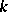be its algebraic closure. A subsetof the Cartesian productis said to be an affine algebraic-set if its points are the common zeros of some familyof the ring of polynomials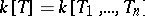. The set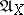of all polynomials in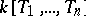that vanish onforms an ideal, the so-called ideal of the affine algebraic-set. The idealcoincides with the radical of the ideal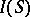generated by the family, i.e. with the set of polynomialssuch that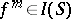for some natural number(Hilbert's Nullstellensatz; cf. Hilbert theorem 3)). Two affine algebraic setsandcoincide if and only if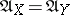. The affine algebraic setcan be defined by a system of generators of. In particular, any affine algebraic set can be defined by a finite number of polynomials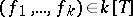. The equalities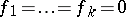are called the equations of. The affine algebraic sets of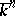form a lattice with respect to the operations of intersection and union. The ideal of the intersection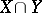is identical with the sum of their ideals, while the ideal of the unionis identical with the intersection of their ideals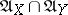. Any setis an affine algebraic set, called an affine space overand denoted by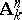; to it corresponds the zero ideal. The empty subset ofis also an affine algebraic set with the unit ideal. The quotient ringis called the coordinate ring of. It is identical with the ring of-regular functions on, i.e. with the ring of-valued functions,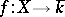, for which there exists a polynomialsuch that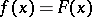for all. An affine algebraic set is said to be irreducible if it is not the union of two affine algebraic proper subsets. An equivalent definition is that the idealis prime. Irreducible affine algebraic sets together with projective algebraic sets were the subjects of classical algebraic geometry. They were called, respectively, affine algebraic varieties and projective algebraic varieties over the field(or-varieties). Affine algebraic sets have the structure of a topological space. The affine algebraic subsets are the closed sets of this topology (the Zariski topology). An affine algebraic set is irreducible if and only if it is irreducible as a topological space. Further development of the concept of an affine algebraic set leads to the concepts of an affine variety and an affine scheme.